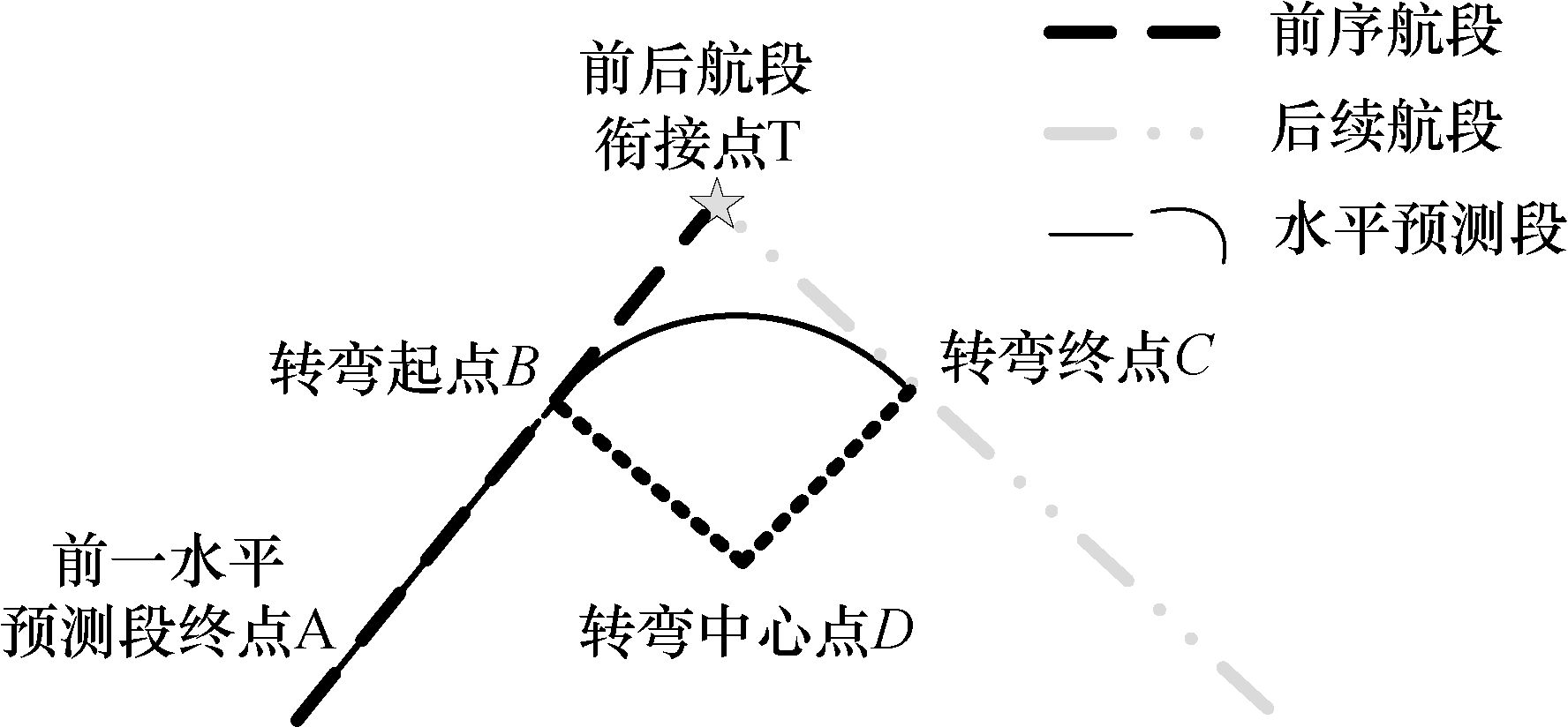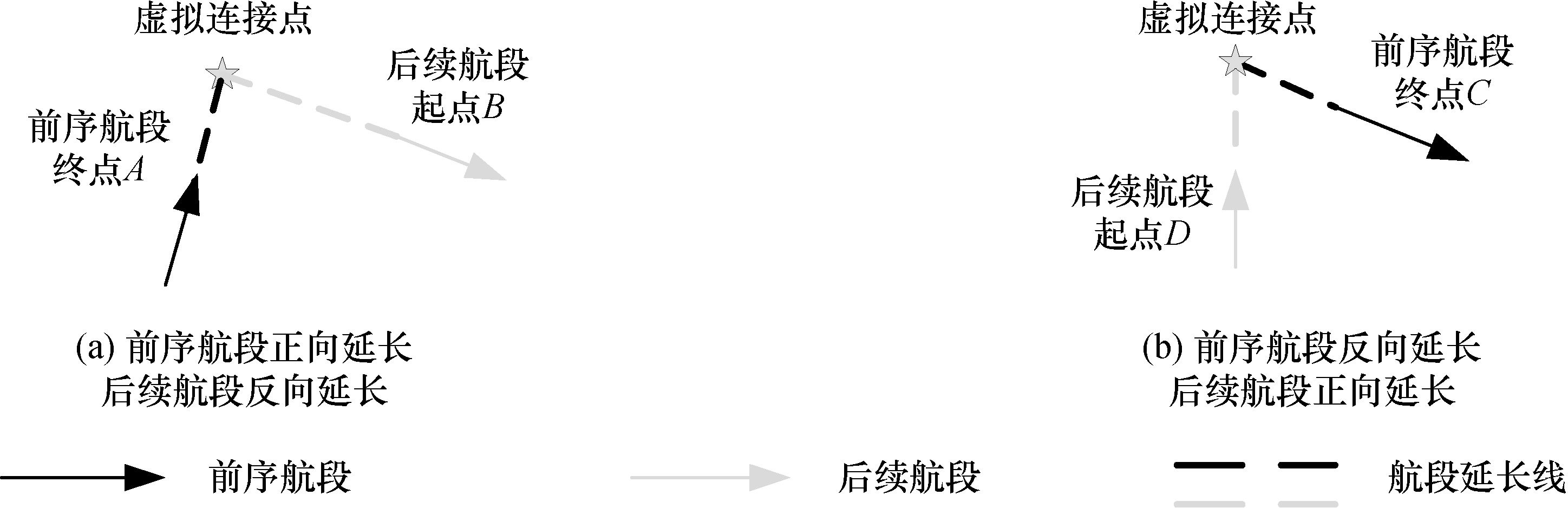1. 清华大学 电子工程系, 北京 100084 ;
2. 中国电子科技集团公司 电子科学研究院, 北京 100041

Optimizing power consumption in distributed antenna systems with large-scale CSIT
WANG Jing1 , WANG Yanmin2 , FENG Wei1 , XIAO Limin1 , ZHOU Shidong11. Department of Electronic Engineering, Tsinghua University, Beijing 100084, China ;
2. Academy of Electronics and Information Technology, China Electronics Technology Group Corporation, Beijing 100041, China
Abstract:The power consumption in distributed antenna systems (DAS) is reduced for green mobile communications by optimizing the total power consumption in downlink DAS with only large-scale channel state information at the transmitter (CSIT) using a practical power consumption model including both the transmission power and the circuit power. The scheme combines transmit antenna selection and power allocation, decomposes the original problem into a series of sub-problems, each of which can be solved by efficient algorithms. The result is a low-complexity joint ordering selection and power allocation algorithm. Simulations show that the total power consumption is reduced considerably by this algorithm while satisfying the ergodic capacity requirements of the users. Thus, this scheme provides practical performance enhancement for DAS with low system overhead.
Key words: mobile communications     distributed antenna systems (DAS)     circuit power consumption     large-scale channel state information at the transmitter (CSIT)

1 系统模型 1.1 DAS系统模型图 1 分布式天线随机放置的DAS系统结构

 $~y=H{{P}_{T}}^{^{1/2}}x+n.$ (1)

 $H={{H}_{\omega }}L.$ (2)

1.2 功耗模型

 $~{{P}_{total}}={{P}_{tx}}/\eta +{{P}_{c}}.$ (3)

 ${{P}_{tx}}=\sum\limits_{k\in K}{{{P}_{k}}}$ (4)

2 问题描述

 ${{E}_{H}}_{\omega }\left[ lbdet\left( {{I}_{M}}+\frac{1}{{{\sigma }_{n}}^{2}}{{H}_{\omega }}L{{P}_{T}}{{H}_{\omega }}{{L}^{H}} \right) \right].$ (5)

 \begin{align} & \underset{K\in N,{{\left\{ {{P}_{k}} \right\}}^{K}}_{k=1}}{\mathop{min}}\,\left( {{P}_{total}}=\sum\limits_{k\in K}{{{P}_{k}}/\eta +K{{P}_{ct}}+{{P}_{co}}} \right), \\ & s.t.\text{ }C\ge {{C}_{min}}. \\ \end{align} (6)

3 基于大尺度CSIT的联合排序和功率分配 3.1 问题分析

 \begin{align} & ({{K}^{*}},{{\{{{P}_{k}}^{*}\}}_{k=1}}^{K})=arg\underset{K\in N|K|=K,{{\{{{P}_{k}}\}}_{k=1}}^{K}}{\mathop{min}}\,{{P}_{total}}, \\ & s.t.\text{ }C\ge {{C}_{min}}. \\ \end{align} (7)

4 仿真验证和分析图 2 不同方案的系统功耗性能对比

5 结 论

  Han C, Harrold T, Armour S, et al. Green radio:radio techniques to enable energy-efficient wireless networks[J]. IEEE Commun Mag , 2011, 49 (6) : 46–54. DOI:10.1109/MCOM.2011.5783984  FENG Wei, GE Ning, LU Jianhua. Hierarchical transmission optimization for massively dense distributed antenna systems[J]. IEEE Commun Lett , 2015, 19 (4) : 673–676. DOI:10.1109/LCOMM.2015.2401584  FENG Wei, WANG Yanmin, GE Ning, et al. Virtual MIMO in multi-cell distributed antenna systems:coordinated transmissions with large-scale CSIT[J]. IEEE J Sel Areas Commun , 2013, 31 (10) : 2067–2081. DOI:10.1109/JSAC.2013.131009  FENG Wei, WANG Yanmin, GE Ning, et al. Adaptive inter-cell coordination for the distributed antenna system with correlated antenna-clusters[C]//Proc ICC 2014. Sydney, Australia:IEEE Press, 2014:5567-5572.  FENG Wei, LI Yunzhou, GAN Jiansong, et al. On the deployment of antenna elements in generalized multi-user distributed antenna systems[J]. Mobile Netw Appl , 2011, 16 (1) : 35–45. DOI:10.1007/s11036-009-0214-1  Cui S G, Goldsmith A J, Bahai A. Energy-efficiency of MIMO and cooperative MIMO techniques in sensor networks[J]. IEEE J Sel Areas Commun , 2004, 22 (6) : 1089–1098. DOI:10.1109/JSAC.2004.830916  He C, Li G Y, Zheng F, et al. Energy-efficient resource allocation in OFDM systems with distributed antennas[J]. IEEE Trans Veh Technol , 2014, 63 (3) : 1223–1231. DOI:10.1109/TVT.2013.2282373  Jiang C, Cimini L J. Antenna selection for energy-efficient MIMO transmission[J]. IEEE Wireless Commun Lett , 2012, 1 (6) : 577–580. DOI:10.1109/LWC.5962382  Li Y, Sheng M, Zhang Y, et al. Energy-efficient antenna selection and power allocation in downlink distributed antenna systems:a stochastic optimization approach[C]//Proc ICC 2014. Sydney, Australia:IEEE Press, 2014:4963-4968.  Wang Y, Feng W, Xiao L, et al. Coordinated multi-cell transmission for distributed antenna systems with partial CSIT[J]. IEEE Commun Lett , 2012, 16 (7) : 1044–1047. DOI:10.1109/LCOMM.2012.050912.120383  FENG Wei, ZHANG Xiujun, ZHOU Shidong, et al. Downlink power allocation for distributed antenna systems with random antenna layout[C]//Proc VTC 2009-Fall. Anchorage, AK, USA:IEEE Press, 2009:1-5.  WANG Tan, WANG Ying. Joint resource allocations in distributed antenna systems with coherent transmitter[C]//Proc GLOBECOM Workshops 2011. Houston, TX, USA:IEEE Press, 2011:194-198.  Liu A, Lau V K N. Joint power and antenna selection optimization for energy-efficient large distributed MIMO networks[C]//Proc ICCS 2012. Singapore:IEEE Press, 2012:230-234. http://digital-library.theiet.org/content/journals/10.1049/iet-map.2014.0714?fmt=bib  Telatar E. Capacity of multi-antenna Gaussian channels[J]. Eur Trans Telecomm , 1999, 10 (6) : 585–596. DOI:10.1002/ett.v10:6  Boyd S, Vandenberghe L. Convex Optimization[M]. Cambridge, UK: Cambridge Univ Press, 2004 .